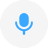• # 加减乘除• [jiā jiǎn chéng chú]

### 释义

• add, subtract, multiply and divide
• ### 实用场景例句

• 全部

Mum: Have you learned addition , subtraction, multiplication , and division, Lucy?妈妈: 露西,你学了加减乘除了 吗 ?

互联网

Addition , subtraction, multiplication and division are all basic operations.加减乘除是最基本的运算法则.

互联网

Addition and subtraction multiplication and division, operator you make endless dedication!加减乘除, 算不尽您作出的奉献!

互联网

Key algorithm can be custom calculation , the number of encryption for every compiler.密匙算法可以通过自定义的加减乘除, 对每一位数字加密编译.

互联网

They often learn addition, subtraction, multiplication and division with the decimal system and with fractions , simultaneously.他们同时还学十进制的加减 乘除 以及小数.

互联网

MCS - 51 single - chip utility subroutine library, addition and subtraction multiplication and division, hexadecimal conversion, floating - point arithmetic, everything!MCS-51 单片机 实用子程序库, 加减乘除, 进制转换, 浮点 算法, 应有尽有!

互联网

Guai guar is quite talented ; she can recognize words and even do some simple calculations. Ms.乖乖会的东西还不只如此, 她不但能认字还能算一些简单的加减乘除的算数.

互联网

Calculator functions to achieve simple calculation, multiplication and division addition and subtraction functions.计算器实现简单计算功能, 加减乘除等功能.

互联网

收起实用场景例句